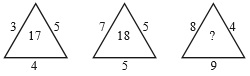# User Forum

Subject :NCO    Class : Class 5

Find the missing number, if a certain rule is followed in the given figures.A23
B33
C28
D41

What is the relation of these figures

## Ans 1:

Class : Class 6
Ans C because in first multiply 5 and 4 and subtract 3 and in other the same pattern goes on . HAPPY LEARNING Thanks

## Ans 2:

Class : Class 6
c the relation in the first one is 5*4=20-3=17 which applies for all

Class : Class 5
C

## Ans 4:

Class : Class 5
C .28= 9*4-8 17=5*4-3 18=5*5-7